Appendix A: A More Detailed Look at the Mathematics

As a vehicle for exploring the mathematics behind STELLA models in more detail, we consider a familiar model - a water tub with a faucet and a drain. Our goal here is to find what is called an anlaytical solution to the question of how much is in the water tub at any time given the basic system structure discussed above and represented mathematically by the equation: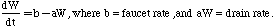We also can specify the initial condition of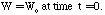This is a classic type of first-order, linear differential equation and the general strategy here is to separate the variables W and t and then integrate both sides. If we do this, the first equation we get is: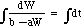(2)

The problem with this is that you can't solve these integrals, so we use a trick, which is to multiply both sides by -a, giving us: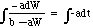(3).

This is a good trick because now the left-hand side of the equation has the form of: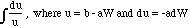This brings us to the point where we can solve both of the integrals, giving: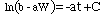(4)

We need to manipulate this a bit further so that we can express it as a function of W. If we remember that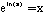then we see that by aplying the natural exponential function to both sides helps us toward our goal of expressing this equation as a function of W: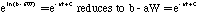(5)

Another trick here is that ex+y = exey and since eC is a constant, which we will call K, we can rewrite the right side of the equation as: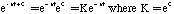Now, we need to find out what K is, and we get help is we look at the initial condition, when t=0, at which time W=W0. Since e0=1, we see that at t=0,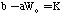Substituting this in to equation (5) gives: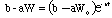Now with just a bit more reordering, we will have an expression for W that works for any time t. First we send b to the right side of the equation, then divide both sides by a, giving: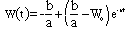(6).

This is our final solution. Having seen this model run, we know that starting at the intial value, the reservoir changes until it reaches a steady state. At that steady state, the inflow is equal to the outflow, which means: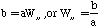This is a nice result since it tells us right away, before the model even runs, the ending steady state value for the water tub.

You might ask, after going through the above math, why do we need a program like STELLA to deal with the differential equations that represent dynamic systems? Why not just use a simple calculator? The reason is that once you move from a simple one-reservoir system to more complex, larger systems, the analytic solution becomes rapidly more complex and eventually impossible.